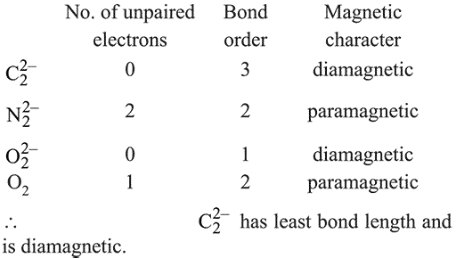# Among the following molecules/ions,Question:

Among the following molecules/ions,

$\mathrm{C}_{2}^{2-}, \mathrm{N}_{2}^{2-}, \mathrm{O}_{2}^{2-}, \mathrm{O}_{2}$

Which one is diamagnetic and has the shortest bond length?

1. $\mathrm{O}_{2}$

2. $\mathrm{N}_{2}^{2-}$

3. $\mathrm{O}_{2}^{2-}$

4. $\mathrm{C}_{2}^{2-}$

Correct Option: , 4

Solution:

Bond length $\propto \frac{1}{\text { Bond order }}$

and diamagnetic species has no unpaired electron in their molecular orbitals.